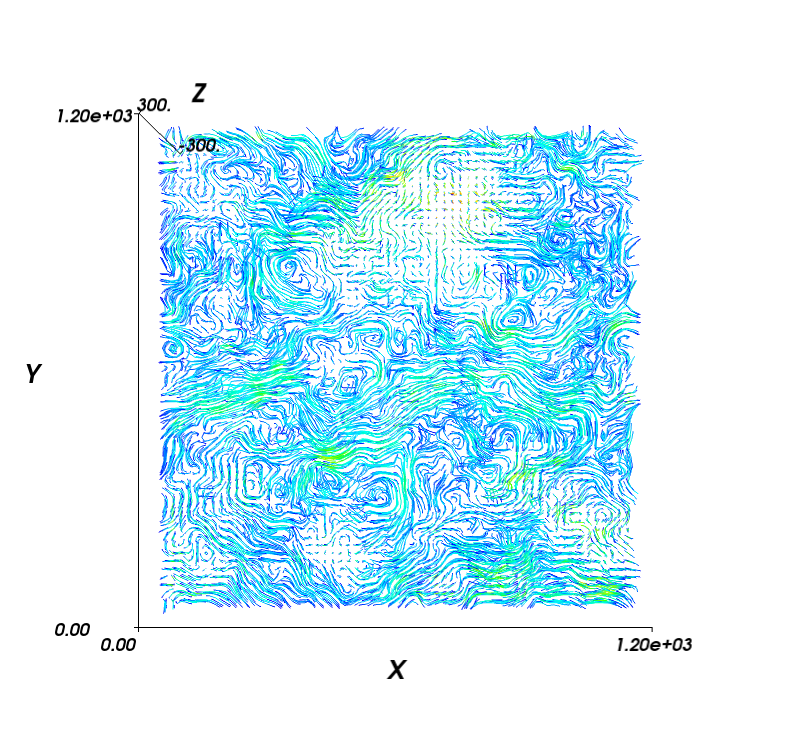# 12.1.2. Streamplots (3D) — `MDAnalysis.visualization.streamlines_3D`

Authors

Tyler Reddy and Matthieu Chavent

Year

2014

The `generate_streamlines_3d()` function can generate a 3D flow field from a MD trajectory, for instance, lipid molecules in a virus capsid. It can make use of multiple cores to perform the analyis in parallel (using `multiprocessing`).

ᵇChavent2014

Matthieu Chavent, Tyler Reddy, Joseph Goose, Anna Caroline E. Dahl, John E. Stone, Bruno Jobard, and Mark S. P. Sansom. Methodologies for the analysis of instantaneous lipid diffusion in md simulations of large membrane systems. Faraday Discuss., 169:455–475, 2014. doi:10.1039/C3FD00145H.

MDAnalysis.visualization.streamlines_3D.generate_streamlines_3d(topology_file_path, trajectory_file_path, grid_spacing, MDA_selection, start_frame, end_frame, xmin, xmax, ymin, ymax, zmin, zmax, maximum_delta_magnitude=2.0, num_cores='maximum')[source]

Produce the x, y and z components of a 3D streamplot data set.

Parameters
• topology_file_path (str) – Absolute path to the topology file

• trajectory_file_path (str) – Absolute path to the trajectory file. It will normally be desirable to filter the trajectory with a tool such as GROMACS g_filter (see )

• grid_spacing (float) – The spacing between grid lines (angstroms)

• MDA_selection (str) – MDAnalysis selection string

• start_frame (int) – First frame number to parse

• end_frame (int) – Last frame number to parse

• xmin (float) – Minimum coordinate boundary for x-axis (angstroms)

• xmax (float) – Maximum coordinate boundary for x-axis (angstroms)

• ymin (float) – Minimum coordinate boundary for y-axis (angstroms)

• ymax (float) – Maximum coordinate boundary for y-axis (angstroms)

• maximum_delta_magnitude (float) – Absolute value of the largest displacement tolerated for the centroid of a group of particles ( angstroms). Values above this displacement will not count in the streamplot (treated as excessively large displacements crossing the periodic boundary)

• num_cores (int or 'maximum' (optional)) – The number of cores to use. (Default ‘maximum’ uses all available cores)

Returns

• dx_array (array of floats) – An array object containing the displacements in the x direction

• dy_array (array of floats) – An array object containing the displacements in the y direction

• dz_array (array of floats) – An array object containing the displacements in the z direction

Examples

Generate 3D streamlines and visualize in mayavi:

```import numpy as np

import MDAnalysis
import MDAnalysis.visualization.streamlines_3D

import mayavi, mayavi.mlab

# assign coordinate system limits and grid spacing:
x_lower,x_upper = -8.73, 1225.96
y_lower,y_upper = -12.58, 1224.34
z_lower,z_upper = -300, 300
grid_spacing_value = 20

x1, y1, z1 = MDAnalysis.visualization.streamlines_3D.generate_streamlines_3d(
'testing.gro', 'testing_filtered.xtc',
xmin=x_lower, xmax=x_upper,
ymin=y_lower, ymax=y_upper,
zmin=z_lower, zmax=z_upper,
grid_spacing=grid_spacing_value, MDA_selection = 'name PO4',
start_frame=2, end_frame=3, num_cores='maximum')

x, y, z = np.mgrid[x_lower:x_upper:x1.shape*1j,
y_lower:y_upper:y1.shape*1j,
z_lower:z_upper:z1.shape*1j]

# plot with mayavi:
fig = mayavi.mlab.figure(bgcolor=(1.0, 1.0, 1.0), size=(800, 800), fgcolor=(0, 0, 0))
for z_value in np.arange(z_lower, z_upper, grid_spacing_value):
st = mayavi.mlab.flow(x, y, z, x1, y1, z1, line_width=1,
seedtype='plane', integration_direction='both')
st.streamline_type = 'tube'